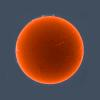# Create a line from randomly numbered points

## Recommended Posts

Hi All!

I want to create a line from some randomly numbered points (see pic.)

In order to use the Add sop the numbering must be ordered by proximity.

Sort by Spatial Proximity does not work good! The numbering that it gives is ok at some areas and messed up in others.

I cant use the Match topology sop because there isn't any geo I can use for that.

So I guess I have to find an alternative way to do it. Any ideas?

Thanks!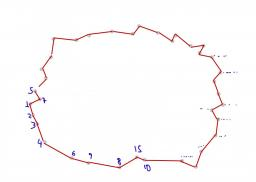##### Share on other sites

If the convex shape of the points is good enough you could triangulate and then remove faces.

Edited by Erik_JE

##### Share on other sites##### Share on other sites

Thank you for you answer guys!

However I'm afraid none of your suggestions works because the points are not flat at all...In the file I actually have to create two lines but I guess if I find a way to create one line out of them I can then delete the first set of points and repeat he procedure on the second set.

I don't think I can do this without somehow "reinventing" the "Sort by Spatial Locality" ...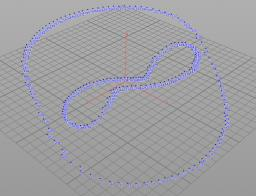Line from points.hipnc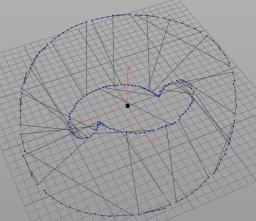##### Share on other sites

There is no easy way of sorting point clouds. I think the only way to do that is to compare points by distance, but this is not always an appropriate solution.

Edited by Stalkerx777

##### Share on other sites

You could create something in a sop solver where you specify an initial point and then crawl from that point to the next based on proximity, while creating a sequential attribute at each iteration. Then you'd sort by attribute.

I don't think any solution will work for every possible case though.

Cheers

Edited by rafaelfs

##### Share on other sites

@Stalkerx777 : " I think the only way to do that is to compare points by distance" ....I totaly agree on that.

I thought that the fact that it is not a"chaotic/noisy" Point Cloud but a rather "tidy/ordered" one, with a visible pattern would make things easier.

@rafelfs: sounds interesting. maybe I will give your suggestion a try later on. I am trying right now to see if the new "Find Shortest Path" node can help somehow...

Edited by dpap

##### Share on other sites

I tried to go another way... I found the nearest point number for each point and tried to make a line using the Foreach sop .

I dont know what am I doing wrong here..

Maybe the whole problem is out of my league for the time being...

foreach nearest.hipnc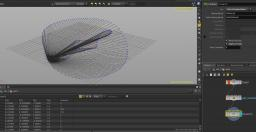##### Share on other sites

I would try to use the attribWrangle node and vex code in combination with a find shortest path node.

I won't be able to provide you with an example right away, but I'll try to describe the way to do it:

There are 2 ways I can think of to do this:

1: This method will work well for the example image of the initial post:

By creating a single step - nearest point connection vex script, and then running the find shortest path over that to order your points.

Use an AttribWrangle and make it do a point cloud operation to store the points within a certain distance to the current point in an array.

Then loop through the array, give every point a temporary variable of VALID = 1.

This loops through the array of points found by the current point.

Now perform checks for each found point using if statements.

If the point number found is the current point being processed set its valid state to 0.

If the point found has a point number lower then the current point set it valid state to 0.

Then using an if statement, push the point numbers of only the valid points into a new array.

Then exit the loop.

Outside the loop, find the point number in the array with the lowest and highest value. Now create a primitive using vex (a two vertex polygon line) between the lowest valid point number and the current point number.

Do the same with the highest valid point number. If a point does not have any valid targets due to the culling, skip it.

Now you will have a system that constructs a maximum of 2 lines per point. This won't be perfect, as it can do branches, but the trick now is to use the start of your curve, (ensure there the shape you just generated is not closed, it has to have a end and start).

Use the find shortest path node's distance function to write out the distance from the start point on your curve.

Then, delete away the primitives, sort the points by this distance attribute and use an add node to connect everything together.

And there you have it, one curve created from your point cloud image.

2: This method is slightly different, and is best suited if you want to create a curve through a curve through a dense point cloud.

you can make a cloud-grid using the nearest point connection vex script described above with some changes. But this one is simpler, simply make it do a connection to every point in range (every point in the point cloud array it finds).

This will connecting all the points to their nearest neighbors.

You can then use the find shortest path node to navigate the grid.

You can do this using way points if you do not need it to navigate every point in your cloud, or using a negative cost per point.

This last method will do the traveling salesman method, where it attempts to pass through every point in your grid at least once.

Edited by hyperforce

##### Share on other sites

You can also just make every point connect to every other point. (in seperate primitives)

then delete the primitives that are too long, fuse, use a connectivity sop+partition sop to divide it into the inner and outer group.

after that you can make both groups form singular primitives by deleting one primitive per shape and using the find shortest path sop to make a single primitive out of it, supplying the start and end point.

then you only need to close both primitives, by using an ends sop for instance.

##### Share on other sites

Hi odForce! Haven't posted on here for years!

I had to solve this exact problem on one of the Spider-Man movies where we needed a way for Spidey's webs to hit surfaces without getting twisted.  We would project points representing the hit position of each thread in the web's terminating 'Eiffel tower' shape.  You get a cloud of points scattered on the surface that isn't necessarily planar. The task is to fit a polygon to the points without getting twists.

So I solved this by automatically calculating the best fit plane through the points and the centroid to give me an axis. Then I sorted the points by polar coordinates around this axis. If you then join the points you get an untwisted polygon. Pretty sure it worked every time.

•6

##### Share on other sites

by disconnecting the start and end of your target curve and then create a path from start to end (the long way around)
finally reconnect the start and end if needed.

alternatively you may want to look at the polypath SOP they added since.

##### Share on other sites

Hey, old thread but figured I'd chime in, if you are looking for something to read only a random nearby point, try this in a wrangle node---
Its smart enough to detect second input geometry. (Pic 1)
Just make sure to hit the `Create Spare Parameters` button on the right of the wrangle node vexpression parameter.
Then set `maxLineLength` and `maxFindCount` up to find near by points using `pcfind`.

```int read=0;
if(npoints(1)>0){
}

int l=len(pts);
float fl=float(l);

int randomConnect=chi("randomConnect");

int rander=pts[int(random(@ptnum*fl+randomConnect)*fl*fl) % l];
vector curPos=attrib(read,"point", "P", rander);

Since I'm not sure the end goal, I'll share this line generator I wrote.  Connects any found points; supports second input detection. (Pic 2)
Same as above, `Create Spare Parameters`
Use `Keep Point Count` to not create needless amounts of duplicate points.

```int drawLine(vector f; vector t){
return line;
}
//-----------------------------

if(npoints(1)>0){
}

int maxLines=chi("maxLineCount");
float minLen=chf("minLineLength");

int randomConnect=chi("randomConnect");
int keepPointCount=min(1, max(0,chi("keepPointCount")));
int runner=0;
vector curPos;
int pt;
if(randomConnect == 0){
for(int x=0; x<len(pts);++x){
pt=pts[x];

if(runner > maxLines){ break; }

if(length(curPos-v@P)>minLen && (@ptnum<pt || read)){
if(keepPointCount){
int to=pt;
}
}else{
drawLine(v@P,curPos);
}
runner++;
}
}
}else{
int l=len(pts);
float fl=float(l);
int rander=pts[int(random(@ptnum*fl+randomConnect)*fl*fl) % l];
if(keepPointCount){
int to=rander;
}
}else{
drawLine(v@P,curPos);
}
}```

Edited by Trancor
Code Fix
•3
•1

##### Share on other sites
On 21/05/2018 at 9:02 AM, Trancor said:

Hey, old thread but figured I'd chime in, if you are looking for something to read only a random nearby point, try this in a wrangle node---
Its smart enough to detect second input geometry. (Pic 1)
Just make sure to hit the `Create Spare Parameters` button on the right of the wrangle node vexpression parameter.
Then set `maxLineLength` and `maxFindCount` up to find near by points using `pcfind`.

```
if(npoints(1)>0){
}

int l=len(pts);
float fl=float(l);

int randomConnect=chi("randomConnect");

int rander=pts[int(random(@ptnum*fl+randomConnect)*fl*fl) % l];
vector curPos=attrib(read,"point", "P", rander);

Since I'm not sure the end goal, I'll share this line generator I wrote.  Connects any found points; supports second input detection. (Pic 2)
Same as above, `Create Spare Parameters`
Use `Keep Point Count` to not create needless amounts of duplicate points.

```
int drawLine(vector f; vector t){
return line;
}
//-----------------------------

if(npoints(1)>0){
}

int maxLines=chi("maxLineCount");
float minLen=chf("minLineLength");

int randomConnect=chi("randomConnect");
int keepPointCount=min(1, max(0,chi("keepPointCount")));
int runner=0;
vector curPos;
int pt;
if(randomConnect == 0){
for(int x=0; x<len(pts);++x){
pt=pts[x];

if(runner > maxLines){ break; }

if(length(curPos-v@P)>minLen && (@ptnum<pt || read)){
if(keepPointCount){
int to=pt;
}
}else{
drawLine(v@P,curPos);
}
runner++;
}
}
}else{
int l=len(pts);
float fl=float(l);
int rander=pts[int(random(@ptnum*fl+randomConnect)*fl*fl) % l];
if(keepPointCount){
int to=rander;
}
}else{
drawLine(v@P,curPos);
}
}```

Fantastic thank you so much for sharing this!

## Create an account or sign in to comment

You need to be a member in order to leave a comment

## Create an account

Sign up for a new account in our community. It's easy!

Register a new account